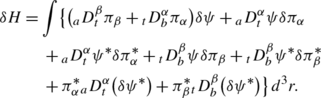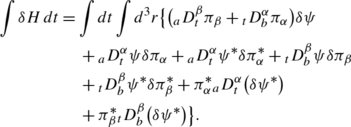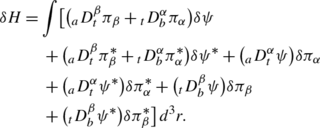# Hamiltonian formulation of classical fields with fractional derivatives: revisited

## Abstract

An investigation of classical fields with fractional derivatives is presented using the fractional Hamiltonian formulation. The fractional Hamilton’s equations are obtained for two classical field examples. The formulation presented and the resulting equations are very similar to those appearing in classical field theory.

This is a preview of subscription content, access via your institution.

## References

1. 1.

Carpinteri A, Mainardi F (1997) Fractals and fractional calculus in continuum mechanics. Springer, Berlin

2. 2.

Novikov VV, Voitsekhovskii KV (2000) Viscoelastic properties of fractal media. J Appl Mech Tech Phys 41:149

3. 3.

Engheta N (1997) On the role of fractional calculus in electromagnetic theory. IEEE Antennas Propag Mag 39:35

4. 4.

Kang-Bo T, Chang-Hong L, Xiao-Jie D (2007) Electrodynamic analysis of dissipative electromagnetic materials based on fractional derivative. Chin Phys Lett 24:847

5. 5.

Gutierrez-Vega JC (2007) Fractionalization of optical beams: I. Planar analysis. Opt Lett 32:1521

6. 6.

Gutierrez-Vega JC (2007) Fractionalization of optical beams: II. Elegant Laguerre-Gauss modes. Opt Express 15:6300

7. 7.

Gaies A, El-Akrmi A (2004) Fractional variational principle in macroscopic picture. Phys Scr 70:7

8. 8.

Hilfer R (ed) (2000) Applications of fractional calculus in physics. World Scientific, Singapore

9. 9.

Oldham KB, Spanier J (2000) The fractional calculus. Academic Press, New York

10. 10.

Podlubny I (1999) Fractional differential equations. Academic Press, New York

11. 11.

Magin RL (2006) Fractional calculus in bioengineering. Begell House, Connecticut

12. 12.

Mainardi F (1994) On the initial problem for the fractional diffusion equation. In: Rionero S, Ruggeri T (eds) Waves and stability in continuous media. World Scientific, Singapore

13. 13.

Mainardi F, Mura A, Pagnini G, Gorenflo R (2007) Sub-diffusion equations of fractional order and their fundamental solutions. In: Tas K, Tenreiro Machado JA (eds) Mathematical methods in engineering. Springer, New York

14. 14.

Metzel R, Klafter J (2000) The random walks guide to anomalous diffusion: a fractional dynamics approach. Phys Rep 339:1–7

15. 15.

Samko SG, Kilbas AA, Marichev OI (1993) Fractional integrals and derivatives—theory and applications. Gordon and Breach, Linghorne

16. 16.

Zaslavsky G (2005) Hamiltonian chaos and fractional dynamics. Oxford University Press, Oxford

17. 17.

West BJ, Bologna M, Grigolini P (2003) Physics of fractal operators. Springer, New York

18. 18.

Agrawal OP (2001) A new Lagrangian and a new Lagrange equation of motion for fractional damped systems. J Appl Mech 53:339

19. 19.

Agrawal OP (1999) An analytical scheme for stochastic dynamics systems containing fractional derivatives. In: ASME design engineering technical conferences

20. 20.

Riewe F (1996) Non conservative Lagrangian and Hamiltonian mechanics. Phys Rev E 53:1890

21. 21.

Riewe F (1997) Mechanics with fractional derivatives. Phys Rev E 55:3581

22. 22.

Jaradat EK, Hijjawi RS, Khalifeh JM (2011) Maxwell’s equations and electromagnetic Lagrangian density in fractional form. J Math Phys 52:033505

23. 23.

Jaradat EK, Hijjawi RS, Khalifeh JM (2010) Fractional canonical quantization of the free electromagnetic Lagrangian density. Jordan J Phys 3:2

24. 24.

Rabei EM, Nawafleh KI, Hijjawi RS, Muslih SI (2007) The Hamiltonian formalism with fractional derivatives. J Math Anal Appl 327:891–897

25. 25.

Samko SG, Kilbas AA, Marichev OI (1993) Fractional integrals and derivatives. Gordon and Breach, Yverdon

26. 26.

Agrawal OP (2002) Formulation of Euler-Lagrange equations for variational problems. J Math Anal Appl 272:368

27. 27.

Schiff L (1968) Quantum mechanics, 3rd edn. McGraw-Hill, New York

28. 28.

Morse P, Feshbach H (1953) Methods of theoretical physics. McGraw-Hill, New York

## Author information

Authors

### Corresponding author

Correspondence to J. M. Khalifeh.

## Appendix: Variation of full Hamiltonian

### Appendix: Variation of full Hamiltonian

We can rewrite Eq. (27) as:

\begin{aligned}[b] H =& \int \bigl[ \pi_{\alpha} {}_{a}D_{t}^{\alpha} \psi+ \pi_{\alpha}^{ *} {}_{a}D_{t}^{\alpha} \psi^{ *} + \pi_{\beta} {}_{t}D_{b}^{\beta} \psi \\ &{}+ \pi_{\beta}^{ *} {}_{t}D_{b}^{\beta} \psi^{ *} \bigr]\, d^{3}r - L. \end{aligned}
(A.1)

Now, take the variation of H, we get:

\begin{aligned}[b] \delta H =& \int\delta\bigl(\pi_{\alpha} {}_{a}D_{t}^{\alpha} \psi+ \pi_{\beta} {}_{t}D_{b}^{\beta} \psi \bigr) \,d^{3}r \\ &{}+ \int\delta\bigl(\pi_{\alpha}^{ *} {}_{a}D_{t}^{\alpha} \psi^{ *} + \pi_{\beta}^{ *} {}_{t}D_{b}^{\beta} \psi^{ *} \bigr) \,d^{3}r - \delta L. \\[6pt] \end{aligned}
(A.2)

Using Eq. (19), Eq. (21) and Eq. (23), we rewrite the variation of full Lagrangian given by Eq. (17) as:

\begin{aligned}[b] \delta L =& \int\bigl\{ - \bigl({}_{a}D_{t}^{\beta} \pi_{\beta} + {}_{t}D_{b}^{\alpha} \pi_{\alpha} \bigr) \delta\psi+ \pi_{\alpha} \delta \bigl({}_{a}D_{t}^{\alpha} \psi\bigr) \\ &{}+ \pi_{\beta} \delta\bigl({}_{t}D_{b}^{\beta} \psi\bigr)\bigr\} \,d^{3}r. \end{aligned}
(A.3)

The above equation can be arranged as:

\begin{aligned}[b] \delta L =& \int\bigl\{ - \bigl({}_{a}D_{t}^{\beta} \pi_{\beta} + {}_{t}D_{b}^{\alpha} \pi_{\alpha} \bigr) \delta\psi \\ &{}+ \delta\bigl(\pi_{\alpha} {}_{a}D_{t}^{\alpha} \psi+ \pi_{\beta} {}_{t}D_{b}^{\beta} \psi\bigr). \\ &{}- {}_{a}D_{t}^{\alpha} \psi\delta\pi_{\alpha} - {}_{t}D_{b}^{\beta} \psi \delta\pi_{\beta} \bigr\} \,d^{3}r. \end{aligned}
(A.4)

Substituting Eq. (A.4) into Eq. (A.2), one gets:(A.5)

Taking the time integration of δH, we get:(A.6)

Integrate (by parts) the last two terms in the above equation then separate integrals over time and integrals over space to get:(A.7)

## Rights and permissions

Reprints and Permissions

Diab, A.A., Hijjawi, R.S., Asad, J.H. et al. Hamiltonian formulation of classical fields with fractional derivatives: revisited. Meccanica 48, 323–330 (2013). https://doi.org/10.1007/s11012-012-9603-9